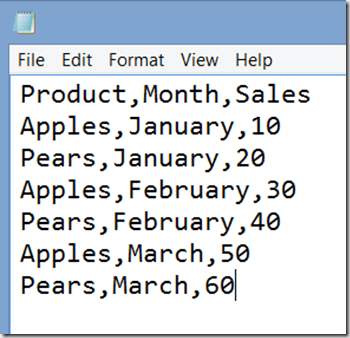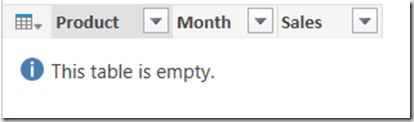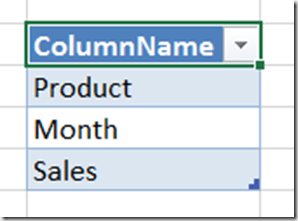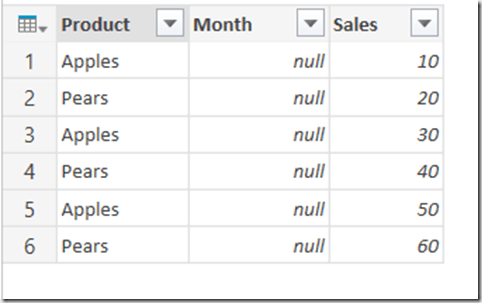# Ensuring Columns Are Always Present In A Table Returned By Power Query

Disappearing or renamed columns in your data source can cause all kinds of problems when you’re importing data using Power Query: errors when you try to refresh the query, broken calculations in Power Pivot, PivotTables that reformat themselves and then need to be manually recreated. As a result, it can be a very good idea to build some logic into your Power Query queries that ensures that a table always contains the columns you’re expecting.

Consider the following csv file:In Power Query, if you connect to it and create a query you’ll end up with something like this:

```let
Source = Csv.Document(File.Contents("C:\Demo.csv"),null,",",null,1252),
#"Changed Type" = Table.TransformColumnTypes(#"First Row as Header",{{"Sales", Int64.Type}})
in
#"Changed Type"
```

Let’s assume that this query is called GetSourceData. Let’s also assume that your output from Power Query should always be a table that has the three columns Product, Month and Sales, and that Product and Month should be text columns and Sales should be numeric. The basic steps to take to ensure that this always happens, even if the columns in the csv file change, are as follows:

1. Create a query that connects to your data source, for example like GetSourceData above
2. Create a query that will always return a table with the columns you want, but which contains no rows
3. Append the second table onto the end of the first table. This will result in a table that contains all of the columns from both tables.
4. Remove any unwanted columns.

There are a number of ways to create the empty table needed in step 2. You could use the #table() function if you’re confident writing M code, and the following single line query (no Let needed) does the job:

```#table(
type table [Product=text, Month=text, Sales=number],
{})
```Alternatively, if you wanted something that an end user could configure themselves, you could start with a table in Excel like this:then transpose it, use the first row of the resulting table as the header row, then set the data types on each table to get the same output:

```let
Source = Excel.CurrentWorkbook(){[Name="Columns"]}[Content],
#"Transposed Table" = Table.Transpose(Source),
#"Changed Type" = Table.TransformColumnTypes(#"First Row as Header",
{{"Product", type text}, {"Month", type text}, {"Sales", Int64.Type}})
in
#"Changed Type"
```

Assuming that this query is called ExpectedColumns, it’s then a trivial task to create a third query that appends the ExpectedColumns query onto the end of the GetSourceData query. If GetSourceData includes all the columns it should then this append will have no effect at all; if some of the columns have changed names or disappeared, you’ll see all of the columns present from both GetSourceData and ExpectedColumns in the output of the append. For example if the Month column in GetSourceData is renamed Months then the output of the append will look like this:Finally, in this third query you need to select all the columns you want (ie all those in the ExpectedColumns query) and right click/Remove Other Columns, so you remove all the columns you don’t want. In the previous example that gives you:The point here is that even though the Month column only contains nulls, and the actual month names have been lost, the fact that the columns are all correct means that you won’t get any errors downstream and your PivotTables won’t be reformatted etc. Once you’ve fixed the problem in the source data and refreshed your queries, everything will go back to normal.

Here’s the code for this third query:

```let
Source = GetSourceData,
Append = Table.Combine({Source,ExpectedColumns}),
#"Removed Other Columns" = Table.SelectColumns(Append,{"Product", "Month", "Sales"})
in
#"Removed Other Columns"
```

For bonus points, here’s another query that compares the columns in GetSourceData and ExpectedColumns and lists any columns that have been added to or are missing from GetSourceData:

```let
//Connect to Excel table containing expected column names
ExcelSource = Excel.CurrentWorkbook(){[Name="Columns"]}[Content],
//Get list of expected columns
ExpectedColumns = Table.Column(ExcelSource, "ColumnName"),
//Get a list of column names in csv
CSVColumns = Table.ColumnNames(GetSourceData),
//Find missing columns
MissingColumns = List.Difference(ExpectedColumns, CSVColumns),
//Report what has changed
OutputMissing = if List.Count(MissingColumns)=0 then
"No columns missing" else
"Missing columns: " & Text.Combine(MissingColumns, ","),
Output = OutputMissing & "   " & OutputAdded
in
Output
```### 10 responses

1.SutoCom says:

Reblogged this on SutoCom Solutions.

2.Curt Hagenlocher says:

I don’t remember if I’ve ever shared anything similar, but you might find the following code useful for this kind of purpose: https://gist.github.com/CurtHagenlocher/806387d0f7bbde3ec4ca

3.milang says:

Maybe not the right place for this comment, but is it possible to multiply two vectors in M (two Lists). It is basic operation and also very common, and very easy to use in R.

I need a validation for one column in a table and this (vector multiplication) would be very useful.

So if I had a value in that column 12345, validation would be something like this
11 – Mod( Sum ( {1,2,3,4,5} * {7,6,5,4,3}), 11)

For now I manage to do this with a function, but it is very slow on large data number.

4.Curt Hagenlocher says:

When you say vector multiplication, I assume you mean the dot product? This can be done relatively efficiently like so:

let List.DotProduct = (a as list, b as list) as number => List.Sum(Table.AddColumn(Table.FromColumns({a, b}), “X”, each [Column1]*[Column2])[X])
in List.DotProduct(List.Random(5000), List.Random(5000))

On my machine, this takes ~10ms.

5.milang says:

Maybe the problem is in another place.
I have a txt file with over 600k rows. This could be the slow part, connection with txt file?
One column in the file contains ID numbers.
I only need to return the rows with valid ID numbers.
ID number has 7 digits. Last digit is used for validating ID number.

Formula for validation in Excel is something like
=Value( Right( [ID_number], 1)) = 11 – MOD( SUMPRODUCT( VALUE( MID( [ID_number], {1,2,3,4,5;6}; 1)), {7,6,5,4,3,2} ), 11)

A valid ID number is 2583518, where validation digit is 8.
VALUE( RIGHT( 2583518, 1)) = 11 – MOD( SUM( {2,5,8,3,5,1} * {7,6,5,4,3,2}), 11)
The sum part is equal to Sum( 2 * 7 + 5 * 6 + 8 * 5 + 3 * 4 + 5 * 3 + 1 * 2) = 113

And I use this PowerQuery function for validating is

let
IdValid = (a as text) as logical =>
// a is column from a table
let
input = a,
s = Text.Start( input, 6),
l = Text.ToList( s),
t1 = Table.FromColumns( { l, {7,6,5,4,3,2} } ),
t2 = Table.TransformColumnTypes( t1, {{“Column1”, Int64.Type}}),
t3 = Table.AddColumn( t2, “X”, each [Column1] * [Column2]),
t4 = List.Sum( t3[X]),
m = 11 – Number.Mod( t4, 11),
f = if m >=10 then 0 else m,
r = Number.FromText( Text.End( input, 1)),
Result = (f = r)
in
Result
in
IdValid

6.milang says:

This function is very slow.
If I dont validate ID column in PQ, it takes around 8 seconds to get the output table in excel, where I can add excel formula for validation.
If I do the validation in PQ it takes around 22 second to get the output table.

Is there a better way to write this validation function in PQ?

7.Curt Hagenlocher says:

This runs in 4 ms on my machine:

let
Validate = (id as text) as logical =>
let
l = List.Transform(Text.ToList(id), each Character.ToNumber(_) – 48),
sum = l{0} * 7 + l{1} * 6 + l{2} * 5 + l{3} * 4 + l{4} * 3 + l{5} * 2
in
11 – Number.Mod(sum, 11) = l{6},
Valid = Validate(“2583518”)
in
Valid

8.Curt Hagenlocher says:

Of course, your version runs in 6 ms, so it’s not exactly a tremendous difference.

This site uses Akismet to reduce spam. Learn how your comment data is processed.